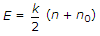# Civil Engineering - Elements of Remote Sensing

### Exercise :: Elements of Remote Sensing - Section 3

6.

"All bodies at temperatures above absolute zero degree emit electromagnetic radiation at different wave length", is known as:

 A. Plank's law B. Planktan's law C. Lambert's cosine law D. None of these

Explanation:

No answer description available for this question. Let us discuss.

7.

Assuming n as the frequency of radiation, n0 as the threshold frequency and k as a constant, the energy of the photo-electrons (E) is related as

 A. E = k (n - n0) B. E = k (n + n0) C. E = k (n x n0) D.Explanation:

No answer description available for this question. Let us discuss.

8.

In which part of the reflective optical infrared region, the effect of multiple leaves in vegetation canopy is maximum ?

 A. The near-IR (0.7 - 1.3 μm) region B. The visible (0.4 - 0.7 μm) region C. The short wave IR (1.3 - 2.7 μm) region D. In all the above region

Explanation:

No answer description available for this question. Let us discuss.

9.

Which one of the following statements is correct ?

 A. The ratio of the permitivity of a medium and the free space, is called relative permitivity. B. Dielectric constant is a pure number and has no dimensions. C. The dielectric constant of pure water is 80. D. Higher the dielectric constant of a medium, the poorer is its electric conductivity. E. All of these

Explanation:

No answer description available for this question. Let us discuss.

10.

The energy transported by the electromagnetic wave is proportional to :

 A. the altitude of the wave B. the square of the altitude of the wave C. the cube of the altitude of the wave D. the square roof of the amplitude